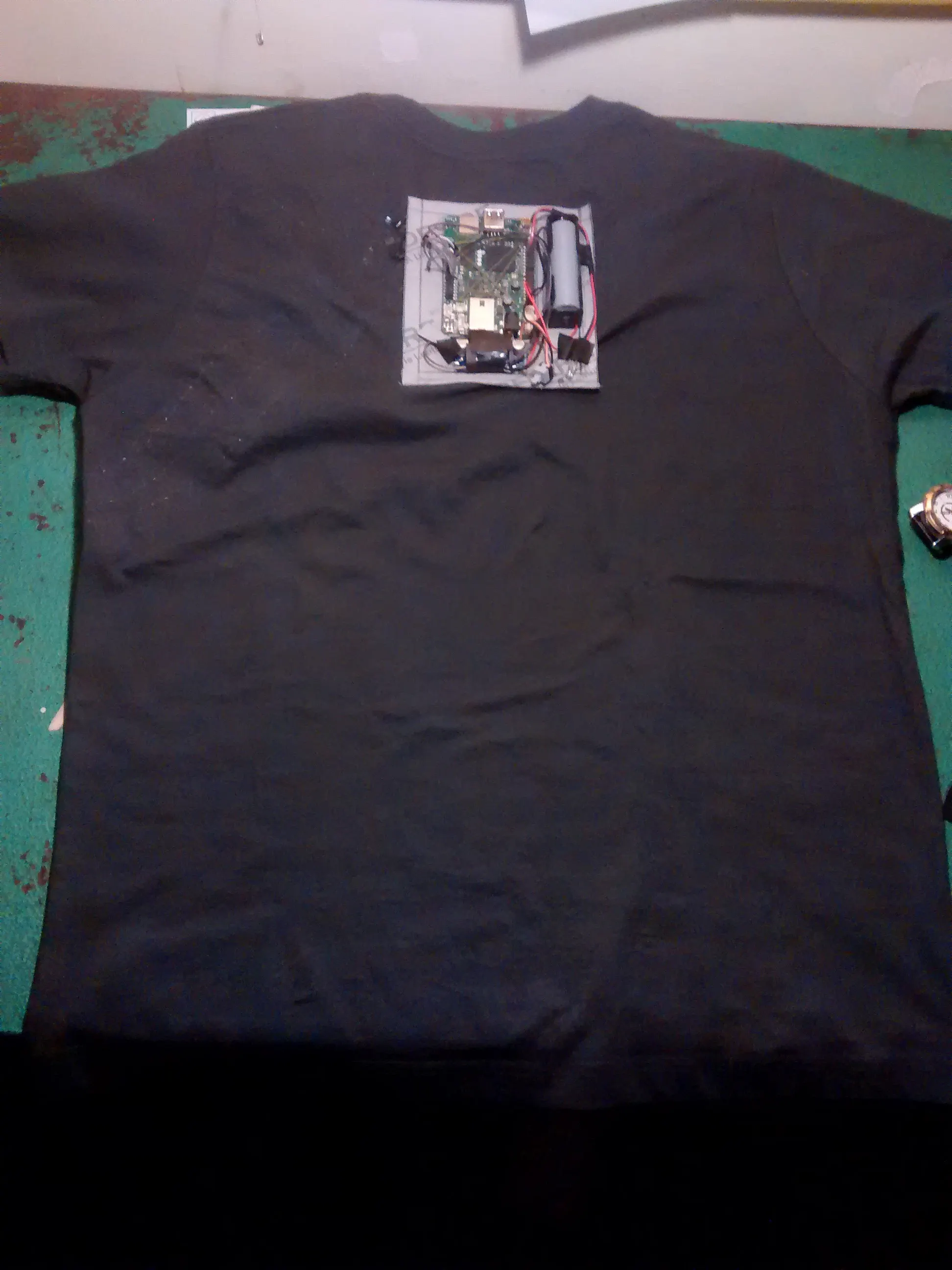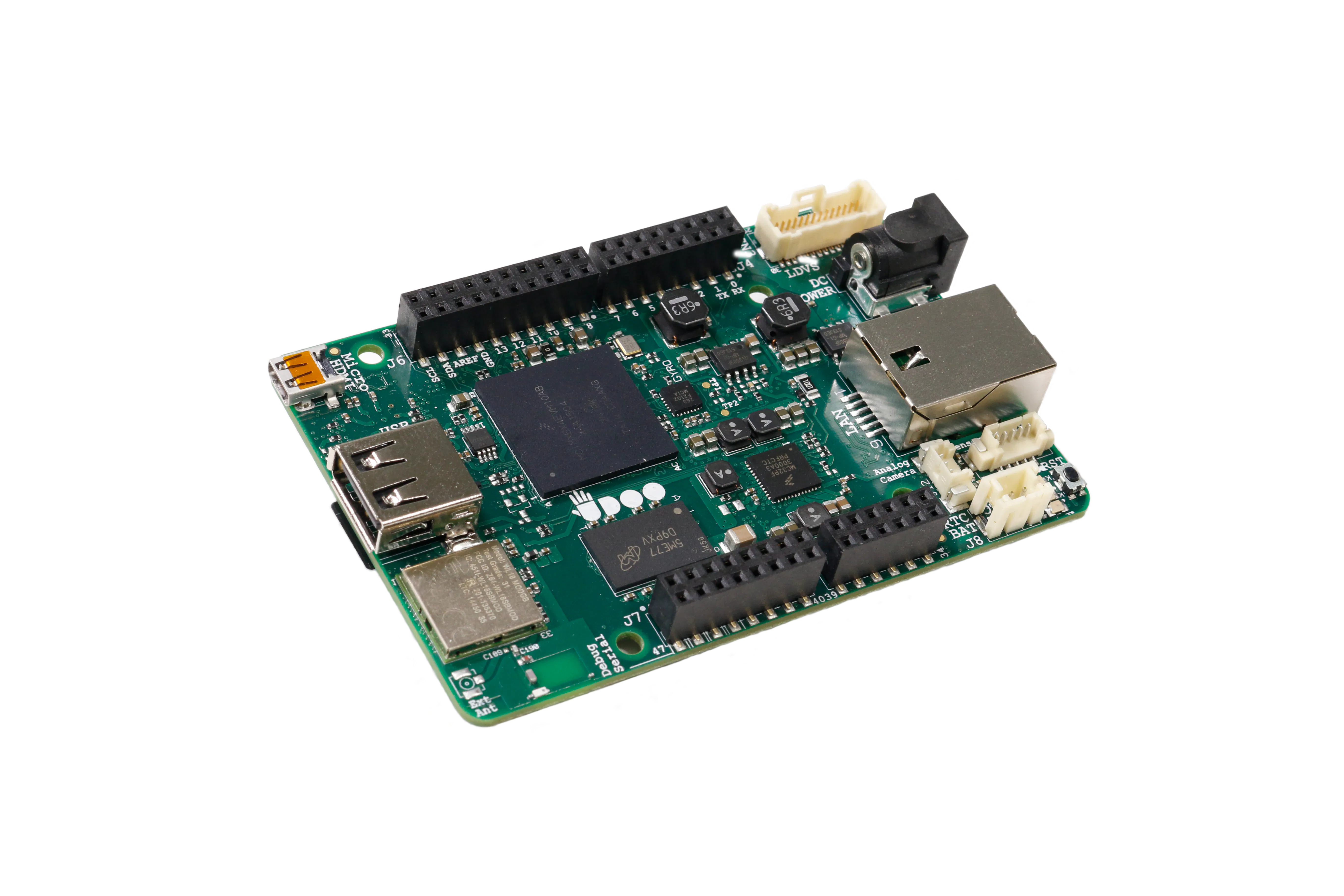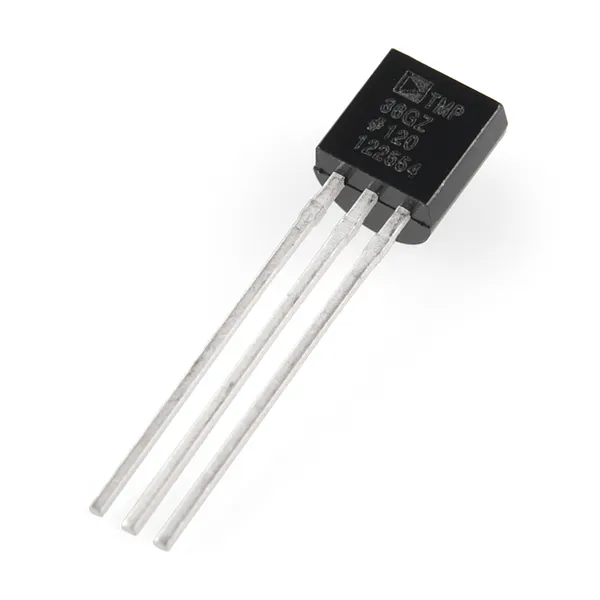Published

# SFAC

A Smart T-shirt designed for athlete and bikers.## Things used in this project

### Hardware componentsUDOO NEO
×1Temperature Sensor
×1
 Heart pulse sensor
×1
 XL6009
×1

### Software apps and online servicesThingSpeak API

### Hand tools and fabrication machines3D Printer (generic)

## Custom parts and enclosures

### Shell

Case for hardware

## Code

### sfacbeta1.5.py

Python
This just the start. We are working on IPC.
```from neo import easyGpio 	    # LED Beacon
from neo import Accel 			# import accelerometer
from neo import Magno 			# import magnometer
from neo import Gyro 				# import gyroscope
from time import sleep			#delay element
#import request					#internet posting request
import httplib, urllib

beacon = easyGpio(13)
beacon.pinOUT() # Make pin output

#motion sensor
gyro = Gyro()
accel = Accel()
magno = Magno()

#Heart pulse tuning
def heartpulse():
global x
if a<=36:
x=73
elif 36<a and a<=72:
x=74
elif 72>a and a<=108:
x=75
elif 108>a and a<=1144:
x=76
elif 144>a and a<=180:
x=77
elif 180>a and a<=216:
x=78
elif 216>a and a<=252:
x=79
elif 252>a and a<=288:
x=80
elif 288>a and a<=324:
x=81
elif 324>a and a<=360:
x=82
elif 360>a and a<=396:
x=83
elif 396>a and a<=432:
x=84
elif 432>a and a<=468:
x=85
elif 468>a and a<=504:
x=86
elif 504>a and a<=540:
x=87
elif 540>a and a<=576:
x=88
elif 576>a and a<=612:
x=89
elif 612>a and a<=648:
x=90
elif 648>a and a<=684:
x=91
elif 684>a and a<=720:
x=92
elif 720>a and a<=756:
x=93
elif 756>a and a<=792:
x=94
elif 792>a and a<=828:
x=95
elif 828>a and a<=864:
x=96
elif 864>a and a<=900:
x=97
elif 900>a and a<=936:
x=98
elif 936>a and a<=972:
x=99
elif 972>a and a<=1008:
x=100
elif 1008>a and a<=1044:
x=101
elif 1044>a and a<=1080:
x=102
elif 1080>a and a<=1116:
x=103
elif 1116>a and a<=1152:
x=104
elif 1152>a and a<=1188:
x=105
elif 1188>a and a<=1224:
x=106
elif 1224>a and a<=1260:
x=107
elif 1260>a and a<=1296:
x=108
elif 1296>a and a<=1332:
x=109
elif 1332>a and a<=1386:
x=110
elif 1386>a and a<=1422:
x=111
elif 1422>a and a<=1458:
x=112
elif 1458>a and a<=1494:
x=113
elif 1494>a and a<=1530
x=114
elif 1530>a and a<=1566:
x=115
elif 1566>a and a<=1602:
x=116
elif 1602>a and a<=1638:
x=117
elif 1638>a and a<=1674:
x=118
elif 1674>a and a<=1710:
x=119
elif 1710>a and a<=1746:
x=120
elif 1746>a and a<=1782:
x=121
elif 1782>a and a<=1818:
x=122
elif 1818>a and a<=1854:
x=123
elif 1854>a and a<=1890:
x=124
elif 1890>a and a<=1926:
x=125
elif 1926>a and a<=1962:
x=126
elif 1962>a and a<=1998:
x=127
elif 1998>a and a<=2034:
x=128
elif 2034>a and a<=2070:
x=129
elif 2070>a and a<=2106:
x=130
elif 2106>a and a<=2142:
x=131
elif 2142>a and a<=2178:
x=132
elif 2178>a and a<=2214:
x=133
elif 2214>a and a<=2250:
x=134
elif 2250>a and a<=2286:
x=135
elif 2286>a and a<=2322:
x=136
elif 2322>a and a<=2358:
x=137
elif 2358>a and a<=2394:
x=138
elif 2394>a and a<=2430:
x=139
elif 2430>a and a<=2466:
x=140
elif 2466>a and a<=2502:
x=141
elif 2502>a and a<=2538:
x=142
elif 2538>a and a<=2574:
x=143
elif 2574>a and a<=2610:
x=144
elif 2610>a and a<=2646:
x=145
elif 2646>a and a<=2682:
x=146
elif 2682>a and a<=2718:
x=147
elif 2718>a and a<=2754:
x=148
elif 2754>a and a<=2790:
x=149
elif 2790>a and a<=2826:
x=150
elif 2826>a and a<=2862:
x=151
elif 2862>a and a<=2898:
x=152
elif 2898>a and a<=2934:
x=153
elif 2934>a and a<=2970:
x=154
elif 2970>a and a<=3006:
x=155
elif 3006>a and a<=3042:
x=156
elif 3042>a and a<=3078:
x=157
elif 3078>a and a<=3114:
x=158
elif 3114>a and a<=3150:
x=159
elif 3150>a and a<=3186:
x=160
elif 3186>a and a<=3222:
x=161
elif 3222>a and a<=3258:
x=162
elif 3258>a and a<=3294:
x=163
elif 3294>a and a<=3330:
x=164
elif 3330>a and a<=3366:
x=165
elif 3366>a and a<=3402:
x=166
elif 3402>a and a<=3438:
x=167
elif 3438>a and a<=3474:
x=168
elif 3474>a and a<=3510:
x=169
elif 3510>a and a<=3546:
x=170
elif 3546>a and a<=3582:
x=171
elif 3582>a and a<=3618:
x=172
elif 3618>a and a<=3654:
x=173
elif 3654>a and a<=3690:
x=174
elif 3690>a and a<=3726:
x=175
elif 3726>a and a<=3762:
x=176
elif 3762>a and a<=3798:
x=177
elif 3798>a and a<=3834:
x=178
elif 3834>a and a<=3870:
x=179
elif 3870>a and a<=3906:
x=180
elif 3906>a and a<=3942:
x=181
elif 3942>a and a<=3978:
x=182
elif 3978>a and a<=4014:
x=183
elif 4014>a and a<=4050:
x=184
else:
x=185
sleep(0)

#Temperature Sensor Tuning
def temperature():
global y
if temp<=440:
y=94
print "Temp is:"+str(y)
elif temp>440 and temp<=445:
y=95
print "Temp is:"+str(y)
elif temp>445 and temp<=450:
y=96
print "Temp is:"+str(y)
elif temp>450 and temp<=460:
y=97
print "Temp is:"+str(y)
elif temp>460 and temp<=465:
y=98
print "Temp is:"+str(y)
elif temp>465 and temp<=470:
y=99
print "Temp is:"+str(y)
elif temp>470 and temp<=475:
y=100
print "Temp is:"+str(y)
elif temp>475 and temp<=480:
y=101
print "Temp is:"+str(y)
elif temp>480 and temp<=485:
y=102
print "Temp is:"+str(y)
elif temp>485and temp<=495:
y=103
print "Temp is:"+str(y)
elif temp>495 and temp<=505:
y=104
print "Temp is:"+str(y)
elif temp>505 and temp<=512:
y=105
print "Temp is:"+str(y)
sleep(0)

#internet posting
def doit():
print ("doit")

params = urllib.urlencode({'field1': x,'field2': y,'field3':accelVals,'field4':accelVals,'field5':accelVals,'field6':gyroVals,'field7':gyroVals,'field8':gyroVals,'field9':magnoVals,'field10':magnoVals,'field11':magnoVals,'key':'5R638RJU5X8G3I1E'})
conn = httplib.HTTPConnection("api.thingspeak.com:80")
try:
response = conn.getresponse()

print response.status, response.reason
conn.close()

except:
print "connection failed"

sleep(0)

while True:

#Post Temperature to Terminal
temperature()
#Post Heart pulse to Terminal
heartpulse()
print'Heart Pulse:'+str(x)

gyroVals = gyro.get() # Returns a full xyz list [x,y,z] realtime (integers/degrees)
print "Gyroscope X: "+str(gyroVals)+" Y: "+str(gyroVals)+" Z: "+str(gyroVals)# turn current values (ints) to strings

accelVals = accel.get() # Same as gyro return xyz of current displacment force
print "Accelerometer X: "+str(accelVals)+" Y: "+str(accelVals)+" Z: "+str(accelVals)

magnoVals = magno.get() # Above
print "Magnometer X: "+str(magnoVals)+" Y: "+str(magnoVals)+" Z: "+str(magnoVals)

beacon.on()
sleep(0.5)
beacon.off()
sleep(0.5)
#POST DATA
doit()

sleep(1)
```